# Remark on the Hurwitz-Lerch zeta function

## Abstract

Various generalizations of the Hurwitz-Lerch zeta function have been actively investigated by many authors. Very recently, Srivastava presented a systematic investigation of numerous interesting properties of some families of generating functions and their partial sums which are associated with various classes of the extended Hurwitz-Lerch zeta functions. In this paper, firstly, we show that by using the Poisson summation formula, the analytic continuation of the Lerch zeta function can be explained and the functional relation for the Lerch zeta function can be obtained in a very elementary way. Secondly, we present another functional relation for the Lerch zeta function and derive the well-known functional relation for the Hurwitz zeta function from our formula by following the lines of Apostol’s argument.

MSC: 11M99, 33B15, 42A24, 11M35, 11M36, 11M41, 42A16.

## 1 Introduction and preliminaries

The Hurwitz-Lerch zeta function is defined as follows: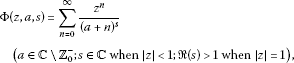(1.1)

where and ${\mathbb{Z}}_{0}^{-}$ denote the set of complex numbers and the set of nonpositive integers, respectively. The function $\mathrm{\Phi }\left(z,a,s\right)$ in (1.1) has been studied in various ways (see, e.g., ). Recently, its generalizations have been investigated (see ). Very recently, Srivastava , motivated essentially by recent works of several authors, presented a systematic investigation of numerous interesting properties of some families of generating functions and their partial sums which are associated with various classes of the extended Hurwitz-Lerch zeta functions (see also the references in ). Here we consider only the case $|z|=1$, i.e., $z={e}^{2\pi ix}$ ($x\in \mathbb{R}$), being the set of real numbers:

$\mathrm{\Phi }\left({e}^{2\pi ix},a,s\right)=\sum _{n=0}^{\mathrm{\infty }}\frac{{e}^{2n\pi ix}}{{\left(a+n\right)}^{s}}\phantom{\rule{1em}{0ex}}\left(\mathrm{\Re }\left(s\right)>1;x\in \mathbb{R};0
(1.2)

It is noted that, for convenience, $\mathrm{\Phi }\left({e}^{2\pi ix},a,s\right)$ in (1.2) is denoted simply by $\mathrm{\Phi }\left(x,a,s\right)$ throughout this paper.

The function $\mathrm{\Phi }\left(x,a,s\right)$ in (1.2) was investigated by Lipschitz [10, 11], Lerch , Apostol [13, 14], and so on. This function $\mathrm{\Phi }\left(x,a,s\right)$ can be extended to the whole s-plane by means of the contour integral

$\mathrm{\Phi }\left(x,a,s\right)=\mathrm{\Gamma }\left(1-s\right)I\left(x,a,s\right),$
(1.3)

where $I\left(x,a,s\right)$ is given by

$I\left(x,a,s\right)=\frac{1}{2\pi i}{\int }_{C}\frac{{z}^{s-1}{e}^{az}}{1-{e}^{z+2\pi ix}}\phantom{\rule{0.2em}{0ex}}dz,$
(1.4)

the path C being a loop which begins at −∞, encircles the origin once in the positive direction, and returns to −∞. Since $I\left(x,a,s\right)$ is an entire function of s, equation (1.3) provides the analytic continuation of $\mathrm{\Phi }\left(x,a,s\right)$. For integer values of x, $\mathrm{\Phi }\left(x,a,s\right)$ reduces to the Hurwitz (or generalized) zeta function $\zeta \left(s,a\right)$ defined by

$\zeta \left(s,a\right)=\sum _{n=0}^{\mathrm{\infty }}\frac{1}{{\left(a+n\right)}^{s}}\phantom{\rule{1em}{0ex}}\left(\mathrm{\Re }\left(s\right)>1;a\in \mathbb{C}\setminus {\mathbb{Z}}_{0}^{-}\right),$
(1.5)

which, by means of (1.3), is a meromorphic function with only a simple pole at $s=1$. For nonintegral x, $\mathrm{\Phi }\left(x,a,s\right)$ becomes an entire function s.

Lerch  presented the functional equation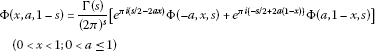(1.6)

by following the lines of the first Riemann proof of the functional equation for the Riemann zeta function :

$\zeta \left(1-s\right)=2{\left(2\pi \right)}^{-s}\mathrm{\Gamma }\left(s\right)cos\left(\frac{\pi s}{2}\right)\zeta \left(s\right)$
(1.7)

and using Cauchy’s theorem in connection with the contour integral (1.4).

Apostol  gave the following functional relation:

$\mathrm{\Lambda }\left(x,a,1-s\right)=2{\left(2\pi \right)}^{-s}cos\left(\frac{\pi s}{2}\right)\mathrm{\Gamma }\left(s\right)exp\left(-2\pi iax\right)\mathrm{\Lambda }\left(-a,x,s\right),$
(1.8)

where

$\mathrm{\Lambda }\left(x,a,s\right):=\mathrm{\Phi }\left(x,a,s\right)+exp\left(-2\pi ix\right)\mathrm{\Phi }\left(-x,1-a,s\right)$
(1.9)

by making a basic use of the transformation theory of theta-functions. Apostol  noted that his proof is of particular interest because the usual approach (Riemann’s second method ) does not lead to the functional equation (1.6) and carried his method through to obtain (1.6) with further properties of $\mathrm{\Phi }\left(x,a,s\right)$, having no analogue in the case of $\zeta \left(s\right)$.

The Hurwitz zeta function (see [16, 17]) defined in (1.5) is given  in the negative half-plane by means of

$\zeta \left(1-s,a\right)=\frac{2\mathrm{\Gamma }\left(s\right)}{{\left(2\pi \right)}^{s}}\sum _{n=1}^{\mathrm{\infty }}\frac{cos\left(\pi s/2-2\pi an\right)}{{n}^{s}}\phantom{\rule{1em}{0ex}}\left(\mathrm{\Re }\left(s\right)>1\right),$
(1.10)

which, upon setting $a=1$, yields the Riemann functional equation (1.7).

Mordell  see also  proved the functional relation for $\zeta \left(s,a\right)$ in (1.10) and that $\zeta \left(s,a\right)$ can be continued meromorphically to the whole s plane except for a simple pole at $s=1$, in a very elementary way, by using Poisson’s summation formula. Apostol  showed that (1.10) could be derived from (1.6) by giving an elaborate argument.

It is pointed out that in order to obtain (1.8), a phrase in the fourth line of [, p.163] ‘replacing s by $1-s$, x by −a, a by x’ should be changed to ‘replacing s by $1-s$, x by a, a by −x’. In fact, if the phrase ‘replacing s by $1-s$, x by −a, a by x’ as it was in [, p.163] is used, the following analogue of (1.8) is obtained:

$\mathrm{\Lambda }\left(x,a,1-s\right)=2{\left(2\pi \right)}^{-s}cos\left(\frac{\pi s}{2}\right)\mathrm{\Gamma }\left(s\right)exp\left(-2\pi iax\right)\mathrm{\Lambda }\left(a,-x,s\right),$
(1.11)

which, upon employing Apostol’s method, yields another functional relation analogous to (1.6):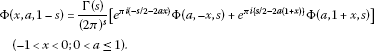(1.12)

Here we aim mainly at, first, showing that $\mathrm{\Phi }\left(x,a,s\right)$ in (1.2) becomes an entire function of s for nonintegral $x\in \mathbb{R}$ and the functional relation for $\mathrm{\Phi }\left(x,a,s\right)$ can be obtained by using Poisson’s summation formula in a very elementary way; and, secondly, deriving the relations (1.11) and (1.12) and showing how the functional relation (1.10) for the Hurwitz zeta function $\zeta \left(s,a\right)$ can be obtained from (1.12) by just following Apostol’s arguments  and , respectively.

## 2 An analytic continuation of $\mathrm{\Phi }\left(x,a,s\right)$

If x is an integer, then $\mathrm{\Phi }\left(x,a,s\right)$ in (1.2) reduces to the Hurwitz zeta function $\zeta \left(s,a\right)$ in (1.5). Since $\mathrm{\Phi }\left(x+1,a,s\right)=\mathrm{\Phi }\left(x,a,s\right)$ for all $x\in \mathbb{R}$, throughout this argument, it is supposed that $\mathrm{\Phi }\left(x,a,s\right)$ is defined at $\mathrm{\Re }\left(s\right)>1$, $0 (x being fixed) and $0.

${\sum _{\alpha }^{\beta }}^{\prime }f\left(n\right)=\sum _{n=-\mathrm{\infty }}^{\mathrm{\infty }}{\int }_{\alpha }^{\beta }{e}^{2n\pi it}f\left(t\right)\phantom{\rule{0.2em}{0ex}}dt,$
(2.1)

where the prime ′ denotes that $n=0$ is omitted from the summation. The summation on the left refers to the integral values of n given by $\alpha \le n\le \beta$; but, when either α or β is an integer, the corresponding term is halved. On the right, the summation means ${lim}_{N\to \mathrm{\infty }}{\sum }_{n=-N}^{N}$. It is supposed that

1. (p)

$f\left(t\right)$ and ${f}^{\prime }\left(t\right)$ are continuous in $\alpha \le t\le \beta$, the obvious one-sided continuity only being required at $t=\alpha$ or $t=\beta$;

2. (q)

$f\left(t\right)$ and ${f}^{″}\left(t\right)$ are such that the integrals ${\int }_{\alpha }^{\beta }f\left(t\right)\phantom{\rule{0.2em}{0ex}}dt$ and ${\int }_{\alpha }^{\beta }{f}^{″}\left(t\right)\phantom{\rule{0.2em}{0ex}}dt$ converge, and ${f}^{\prime }$ is an integral of ${f}^{″}$.

If either α or β is infinite, say $\alpha =-\mathrm{\infty }$, further condition is required that $f\left(t\right)\to 0$ and ${f}^{\prime }\left(t\right)\to 0$ as $t\to \alpha$.

Applying Poisson’s summation formula (2.1) to a function

$f\left(t\right)=\frac{{e}^{2\pi xit}}{{\left(t+a\right)}^{s}},\phantom{\rule{2em}{0ex}}\alpha =0,\phantom{\rule{1em}{0ex}}\text{and}\phantom{\rule{1em}{0ex}}\beta =\mathrm{\infty },$

we find that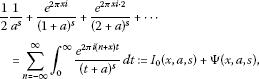(2.2)

where, for convenience, n being an integer,

${I}_{n}\left(x,a,s\right):={\int }_{0}^{\mathrm{\infty }}\frac{{e}^{2\pi i\left(n+x\right)t}}{{\left(t+a\right)}^{s}}\phantom{\rule{0.2em}{0ex}}dt,\phantom{\rule{2em}{0ex}}\mathrm{\Psi }\left(x,a,s\right):=\sum _{\begin{array}{c}n=-\mathrm{\infty }\\ n\ne 0\end{array}}^{\mathrm{\infty }}{I}_{n}\left(x,a,s\right).$
(2.3)

Integrating by parts yields

${I}_{0}\left(x,a,s\right)=-\frac{1}{2\pi xi{a}^{s}}+\frac{s}{2\pi xi}{\int }_{0}^{\mathrm{\infty }}\frac{{e}^{2\pi ixt}}{{\left(t+a\right)}^{s+1}}\phantom{\rule{0.2em}{0ex}}dt.$
(2.4)

It is observed that the integral in (2.4) now converges for $\mathrm{\Re }\left(s\right)>0$, and so ${I}_{0}\left(x,a,s\right)$ is analytic for $\mathrm{\Re }\left(s\right)>0$. Integrating by parts again in (2.4), we get

${I}_{0}\left(x,a,s\right)=-\frac{1}{2\pi xi{a}^{s}}-\frac{s}{{\left(2\pi xi\right)}^{2}{a}^{s+1}}+\frac{s\left(s+1\right)}{{\left(2\pi xi\right)}^{2}}{\int }_{0}^{\mathrm{\infty }}\frac{{e}^{2\pi ixt}}{{\left(t+a\right)}^{s+2}}\phantom{\rule{0.2em}{0ex}}dt.$
(2.5)

It is also observed that the integral in (2.5) now converges $\mathrm{\Re }\left(s\right)>-1$, and so ${I}_{0}\left(x,a,s\right)$ is analytic for $\mathrm{\Re }\left(s\right)>-1$. Continuing in this way, it is found that ${I}_{0}\left(x,a,s\right)$ can be continued to an entire function of s.

Integrating by parts yields

$\mathrm{\Psi }\left(x,a,s\right)=-\frac{1}{2\pi i{a}^{s}}\sum _{\begin{array}{c}n=-\mathrm{\infty }\\ n\ne 0\end{array}}^{\mathrm{\infty }}\frac{1}{n+x}+\frac{s}{2\pi i}\sum _{\begin{array}{c}n=-\mathrm{\infty }\\ n\ne 0\end{array}}^{\mathrm{\infty }}\frac{1}{n+x}{\int }_{0}^{\mathrm{\infty }}\frac{{e}^{2\pi i\left(n+x\right)t}}{{\left(t+a\right)}^{s+1}}\phantom{\rule{0.2em}{0ex}}dt.$
(2.6)

Here we have

$\sum _{\begin{array}{c}n=-\mathrm{\infty }\\ n\ne 0\end{array}}^{\mathrm{\infty }}\frac{1}{n+x}=\underset{N\to \mathrm{\infty }}{lim}\sum _{\begin{array}{c}n=-N\\ n\ne 0\end{array}}^{N}\frac{1}{n+x}=\underset{N\to \mathrm{\infty }}{lim}\sum _{n=1}^{N}\left(\frac{1}{x+n}+\frac{1}{x-n}\right)=2x\sum _{n=1}^{\mathrm{\infty }}\frac{1}{{x}^{2}-{n}^{2}},$
(2.7)

which converges for every fixed x with $0. Likewise, it is seen that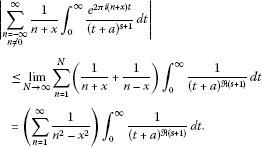(2.8)

It is noted that the last integral in (2.8) converges for $\mathrm{\Re }\left(s\right)>0$, and the second summation in (2.6) converges for $\mathrm{\Re }\left(s\right)>0$. Therefore $\mathrm{\Psi }\left(x,a,s\right)$ in (2.6) is analytic for $\mathrm{\Re }\left(s\right)>0$.

Integrating by parts in (2.6) and considering (2.7), we get

$\begin{array}{rcl}\mathrm{\Psi }\left(x,a,s\right)& =& \left(\frac{x}{\pi i}\sum _{n=1}^{\mathrm{\infty }}\frac{1}{{n}^{2}-{x}^{2}}\right)\frac{1}{{a}^{s}}\\ +\frac{s}{{\left(2\pi i\right)}^{2}}\sum _{\begin{array}{c}n=-\mathrm{\infty }\\ n\ne 0\end{array}}^{\mathrm{\infty }}\frac{1}{{\left(n+x\right)}^{2}}\left\{-\frac{1}{{a}^{s+1}}+\left(s+1\right){\int }_{0}^{\mathrm{\infty }}\frac{{e}^{2\pi i\left(n+x\right)t}}{{\left(t+a\right)}^{s+2}}\phantom{\rule{0.2em}{0ex}}dt\right\}.\end{array}$
(2.9)

The integral in (2.9) converges for $\mathrm{\Re }\left(s\right)>-1$. The expression of $\mathrm{\Psi }\left(x,a,s\right)$ in (2.9), proved for $\mathrm{\Re }\left(s\right)>1$, shows that $\mathrm{\Psi }\left(x,a,s\right)$ is analytic for $\mathrm{\Re }\left(s\right)>-1$ since then the general term is $O\left(1/{n}^{2}\right)$ uniformly in s for s bounded and $-1<-1+\delta \le \mathrm{\Re }\left(s\right)$ for every $\delta >0$. Employing integration by parts repeatedly, we observe that $\mathrm{\Psi }\left(x,a,s\right)$ can be continued analytically to the whole s plane.

It is found from (2.2), (2.3), (2.4), (2.6), and (2.7) that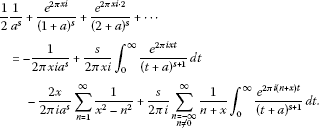(2.10)

Suppose now that $\mathrm{\Re }\left(s\right)<0$. Then Poisson’s summation formula (2.1) gives

$\frac{1}{2}\frac{1}{{a}^{s}}={\int }_{-a}^{0}\frac{{e}^{2\pi xit}}{{\left(t+a\right)}^{s}}\phantom{\rule{0.2em}{0ex}}dt+\sum _{\begin{array}{c}n=-\mathrm{\infty }\\ n\ne 0\end{array}}^{\mathrm{\infty }}{\int }_{-a}^{0}\frac{{e}^{2\pi i\left(n+x\right)t}}{{\left(t+a\right)}^{s}}\phantom{\rule{0.2em}{0ex}}dt,$

which, upon integrating by parts two involved integrals and using (2.7), yields

$\begin{array}{rcl}\frac{1}{2}\frac{1}{{a}^{s}}& =& \frac{1}{2\pi xi{a}^{s}}+\frac{s}{2\pi xi}{\int }_{-a}^{0}\frac{{e}^{2\pi ixt}}{{\left(t+a\right)}^{s+1}}\phantom{\rule{0.2em}{0ex}}dt\\ +\frac{2x}{2\pi i{a}^{s}}\sum _{n=1}^{\mathrm{\infty }}\frac{1}{{x}^{2}-{n}^{2}}+\frac{s}{2\pi i}\sum _{\begin{array}{c}n=-\mathrm{\infty }\\ n\ne 0\end{array}}^{\mathrm{\infty }}\frac{1}{n+x}{\int }_{-a}^{0}\frac{{e}^{2\pi i\left(n+x\right)t}}{{\left(t+a\right)}^{s+1}}\phantom{\rule{0.2em}{0ex}}dt.\end{array}$
(2.11)

Adding (2.10) to (2.11) with the restriction of $-1<\mathrm{\Re }\left(s\right)<0$, we get

$\begin{array}{rcl}\mathrm{\Phi }\left(x,a,s\right)& =& \frac{s}{2\pi i}\sum _{n=-\mathrm{\infty }}^{\mathrm{\infty }}\frac{{e}^{-2\pi ia\left(n+x\right)}}{n+x}{\int }_{0}^{\mathrm{\infty }}\frac{{e}^{2\pi i\left(n+x\right)t}}{{t}^{s+1}}\phantom{\rule{0.2em}{0ex}}dt\\ =& \frac{s}{2\pi i}\left(\sum _{n=0}^{\mathrm{\infty }}+\sum _{n=-\mathrm{\infty }}^{-1}\right)\frac{{e}^{-2\pi ia\left(n+x\right)}}{n+x}{\int }_{0}^{\mathrm{\infty }}\frac{{e}^{2\pi i\left(n+x\right)t}}{{t}^{s+1}}\phantom{\rule{0.2em}{0ex}}dt,\end{array}$

which yields

$\begin{array}{rcl}\mathrm{\Phi }\left(x,a,s\right)& =& \frac{s}{2\pi i}\left[\sum _{n=0}^{\mathrm{\infty }}\frac{{e}^{-2\pi ia\left(n+x\right)}}{n+x}{\int }_{0}^{\mathrm{\infty }}\frac{{e}^{2\pi i\left(n+x\right)t}}{{t}^{s+1}}\phantom{\rule{0.2em}{0ex}}dt\\ -\sum _{n=0}^{\mathrm{\infty }}\frac{{e}^{2\pi ia\left(n+1-x\right)}}{n+1-x}{\int }_{0}^{\mathrm{\infty }}\frac{{e}^{-2\pi i\left(n+1-x\right)t}}{{t}^{s+1}}\phantom{\rule{0.2em}{0ex}}dt\right].\end{array}$
(2.12)

Applying the following integral formulas to (2.12):

${\int }_{0}^{\mathrm{\infty }}\frac{{e}^{it}}{{t}^{s}}\phantom{\rule{0.2em}{0ex}}dt=i{e}^{-\frac{\pi s}{2}i}\mathrm{\Gamma }\left(1-s\right)\phantom{\rule{1em}{0ex}}\left(0<\mathrm{\Re }\left(s\right)<1\right)$
(2.13)

and

${\int }_{0}^{\mathrm{\infty }}\frac{{e}^{-it}}{{t}^{s}}\phantom{\rule{0.2em}{0ex}}dt=-i{e}^{\frac{\pi s}{2}i}\mathrm{\Gamma }\left(1-s\right)\phantom{\rule{1em}{0ex}}\left(0<\mathrm{\Re }\left(s\right)<1\right),$
(2.14)

we obtain, for $-1<\mathrm{\Re }\left(s\right)<0$,

$\begin{array}{rcl}\mathrm{\Phi }\left(x,a,s\right)& =& \frac{\mathrm{\Gamma }\left(1-s\right)}{{\left(2\pi \right)}^{1-s}}\left\{{e}^{\pi i\left(-2ax-\frac{s}{2}+\frac{1}{2}\right)}\sum _{n=0}^{\mathrm{\infty }}\frac{{e}^{-2\pi ian}}{{\left(n+x\right)}^{1-s}}\\ +{e}^{\pi i\left\{2a\left(1-x\right)+\frac{s}{2}-\frac{1}{2}\right\}}\sum _{n=0}^{\mathrm{\infty }}\frac{{e}^{2\pi ian}}{{\left(n+1-x\right)}^{1-s}}\right\}.\end{array}$
(2.15)

It is noted that (2.15) still holds for $\mathrm{\Re }\left(s\right)<0$ since the two involved series converge uniformly in s for $\mathrm{\Re }\left(s\right)\le \delta <0$ (every $\delta <0$). Finally, we get, for $\mathrm{\Re }\left(s\right)<0$,

$\begin{array}{rcl}\mathrm{\Phi }\left(x,a,s\right)& =& \frac{\mathrm{\Gamma }\left(1-s\right)}{{\left(2\pi \right)}^{1-s}}\left[{e}^{\pi i\left(-2ax-\frac{s}{2}+\frac{1}{2}\right)}\mathrm{\Phi }\left(-a,x,1-s\right)\\ +{e}^{\pi i\left\{2a\left(1-x\right)+\frac{s}{2}-\frac{1}{2}\right\}}\mathrm{\Phi }\left(a,1-x,1-s\right)\right],\end{array}$
(2.16)

which, upon replacing s by $1-s$, yields (1.6).

## 3 Proof of (1.11) and (1.12)

We rewrite Apostol’s argument  in a little shorter way. The theta-function

${\vartheta }_{3}\left(y|\tau \right)=\sum _{n=-\mathrm{\infty }}^{\mathrm{\infty }}exp\left(\pi i{n}^{2}\tau +2iny\right)$
(3.1)

has the transformation formula [, p.475]

${\vartheta }_{3}\left(y|\tau \right)={\left(-i\tau \right)}^{-1/2}exp\left(\frac{{y}^{2}}{\pi i\tau }\right){\vartheta }_{3}\left(\frac{y}{\tau }|\frac{-1}{\tau }\right).$
(3.2)

Using (3.2), we have the functional equation

$\theta \left(a,-x,1/z\right)=exp\left(2\pi iax\right){z}^{1/2}\theta \left(x,a,z\right),$
(3.3)

where

$\begin{array}{rcl}\theta \left(x,a,z\right)& :=& exp\left(-\pi {a}^{2}z\right){\vartheta }_{3}\left(\pi x+\pi iaz|iz\right)\\ =& \sum _{n=-\mathrm{\infty }}^{\mathrm{\infty }}exp\left\{2n\pi ix-\pi z{\left(a+n\right)}^{2}\right\}.\end{array}$
(3.4)

Using the key formal identity to Riemann’s second method

${\pi }^{-s/2}\mathrm{\Gamma }\left(\frac{s}{2}\right)\sum _{n=1}^{\mathrm{\infty }}{a}_{n}{f}_{n}^{-s/2}={\int }_{0}^{\mathrm{\infty }}{z}^{s/2-1}\sum _{n=1}^{\mathrm{\infty }}{a}_{n}exp\left(-\pi z{f}_{n}\right)\phantom{\rule{0.2em}{0ex}}dz,$
(3.5)

we get

${\pi }^{-s/2}\mathrm{\Gamma }\left(\frac{s}{2}\right)\mathrm{\Lambda }\left(x,a,s\right)=\left({\int }_{1}^{\mathrm{\infty }}+{\int }_{0}^{1}\right){z}^{s/2-1}\theta \left(x,a,z\right)\phantom{\rule{0.2em}{0ex}}dz,$
(3.6)

where $\mathrm{\Lambda }\left(x,a,s\right)$ is given in (1.9). In the second integral in (3.6), applying (3.3) and replacing z by $1/z$, we have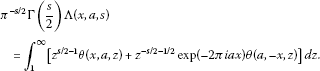(3.7)

Here, if we replace s by $1-s$, x by a, a by −x in (3.7), and use $\theta \left(-a,x,z\right)=\theta \left(a,-x,z\right)$ and the relation

${\pi }^{1/2-s}\mathrm{\Gamma }\left(\frac{s}{2}\right)/\mathrm{\Gamma }\left(\frac{1-s}{2}\right)=2{\left(2\pi \right)}^{-s}cos\left(\frac{\pi s}{2}\right)\mathrm{\Gamma }\left(s\right),$

we are led to the relation (1.8). Instead, replacing s by $1-s$, x by −a, a by x in (3.7) as they were in [, p.163], we obtain the desired identity (1.11).

Now differentiating both sides of (1.1) with respect to a and using the following differential-difference equations satisfied by Φ:

$\frac{\partial \mathrm{\Phi }\left(x,a,s\right)}{\partial a}=-s\mathrm{\Phi }\left(x,a,s+1\right)$
(3.8)

and

$\frac{\partial \mathrm{\Phi }\left(x,a,s\right)}{\partial x}+2\pi ia\mathrm{\Phi }\left(x,a,s\right)=2\pi i\mathrm{\Phi }\left(x,a,s-1\right),$
(3.9)

we obtain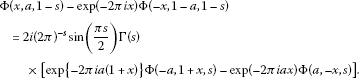(3.10)

Adding (1.11) to (3.10), we are led to the desired relation (1.12).

## 4 (1.10) can be deduced from (1.12)

Apostol  could deduce (1.10) from (1.6) by using an elaborate argument. Here, we also show that (1.10) can be deduced from (1.12) by following the lines of Apostol’s argument. For convenience, we recover the Apostol’s method. Consider the sum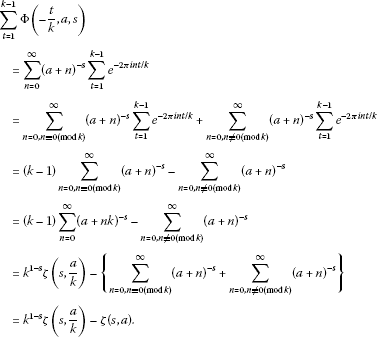The above rearrangements are all valid if $\mathrm{\Re }\left(s\right)>1$ and the final result holds for all s by analytic continuation. Replacing s by $1-s$, we get

$\sum _{t=1}^{k-1}\mathrm{\Phi }\left(-\frac{t}{k},a,1-s\right)={k}^{s}\zeta \left(1-s,\frac{a}{k}\right)-\zeta \left(1-s,a\right)\phantom{\rule{1em}{0ex}}\left(k\in \mathbb{N}\right),$
(4.1)

where the empty sum is understood to be nil.

Now we write $x=-t/k$ in (1.12), assume $\mathrm{\Re }\left(s\right)>1$, and sum on t to obtain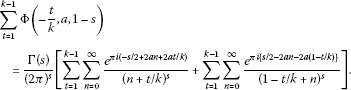Replacing $1-t/k$ by $t/k$ in the second double summation in the brackets does not alter the sum over t, and we obtain

$\sum _{t=1}^{k-1}\mathrm{\Phi }\left(-\frac{t}{k},a,1-s\right)=\frac{2\mathrm{\Gamma }\left(s\right)}{{\left(2\pi \right)}^{s}}\sum _{t=1}^{k-1}\sum _{n=0}^{\mathrm{\infty }}\frac{cos\left\{\frac{\pi s}{2}-2\pi a\left(n+t/k\right)\right\}}{{\left(n+t/k\right)}^{s}}.$

If we write $\lambda =nk+t$, then λ takes on all positive integer values which are not multiples of k as n, t run through their respective ranges, and our sum becomes

$\sum _{t=1}^{k-1}\mathrm{\Phi }\left(-\frac{t}{k},a,1-s\right)=\frac{2\mathrm{\Gamma }\left(s\right){k}^{s}}{{\left(2\pi \right)}^{s}}\sum _{\lambda =1}^{\mathrm{\infty }}\left\{\frac{cos\left(\frac{\pi s}{2}-2\pi a\lambda /k\right)}{{\lambda }^{s}}-\frac{cos\left(\frac{\pi s}{2}-2\pi a\lambda \right)}{{\left(k\lambda \right)}^{s}}\right\}.$
(4.2)

Combining (4.1) and (4.2) yields the equation

${k}^{s}\mathrm{\Delta }\left(s,\frac{a}{k}\right)=\mathrm{\Delta }\left(s,a\right),$
(4.3)

where

$\mathrm{\Delta }\left(s,a\right):=\zeta \left(1-s,a\right)-\frac{2\mathrm{\Gamma }\left(s\right)}{{\left(2\pi \right)}^{s}}\sum _{n=1}^{\mathrm{\infty }}\frac{cos\left(\frac{\pi s}{2}-2\pi an\right)}{{n}^{s}}.$
(4.4)

Now, by repeating Apostol’s argument, it can be shown that $\mathrm{\Delta }\left(s,a\right)$ vanishes identically for $\mathrm{\Re }\left(s\right)>1$.

## References

1. Ferreira C, López J: Asymptotic expansions of the Hurwitz-Lerch zeta function. J. Math. Anal. Appl. 2004, 298: 210–224. 10.1016/j.jmaa.2004.05.040

2. Choi J, Jang DS, Srivastava HM: A generalization of the Hurwitz-Lerch zeta function. Integral Transforms Spec. Funct. 2008, 19: 65–79.

3. Garg M, Jain K, Srivastava HM: Some relationships between the generalized Apostol-Bernoulli polynomials and Hurwitz-Lerch zeta functions. Integral Transforms Spec. Funct. 2006, 17: 803–815. 10.1080/10652460600926907

4. Goyal SP, Laddha RK: On the generalized Riemann zeta functions and the generalized Lambert transform. Ganita Sandesh 1997, 11: 99–108.

5. Lin S-D, Srivastava HM: Some families of the Hurwitz-Lerch zeta functions and associated fractional derivative and other integral representations. Appl. Math. Comput. 2004, 154: 725–733. 10.1016/S0096-3003(03)00746-X

6. Lin S-D, Srivastava HM, Wang P-Y: Some expansion formulas for a class of generalized Hurwitz-Lerch zeta functions. Integral Transforms Spec. Funct. 2006, 17: 817–827. 10.1080/10652460600926923

7. Srivastava HM, Choi J: Series Associated with the Zeta and Related Functions. Kluwer Academic, Dordrecht; 2001.

8. Srivastava HM, Choi J: Zeta and q-Zeta Functions and Associated Series and Integrals. Elsevier, Amsterdam; 2012.

9. Srivastava HM: Generating relations and other results associated with some families of the extended Hurwitz-Lerch zeta functions. SpringerPlus 2013., 2: Article ID 67. doi:10.1186/2193–1801–2-67. http://www.springerplus.com/content/pdf/2193–1801–2-67.pdf

10. Lipschitz R: Untersuchung einer aus vier Elementen gebildeten Reihe. J. Reine Angew. Math. 1857, 54: 313–328.

11. Lipschitz R: Untersuchung der Eigenschaften einer Gattung von unendlichen Reihen. J. Reine Angew. Math. 1889, 105: 127–156.

12. Lerch M:Note sur la fonction $\mathcal{R}\left(w,x,s\right)={\sum }_{k=0}^{\mathrm{\infty }}\frac{{e}^{2k\pi ix}}{{\left(w+k\right)}^{s}}$. Acta Math. 1887, 11: 19–24. 10.1007/BF02612318

13. Apostol TM: On the Lerch zeta function. Pac. J. Math. 1951, 1: 161–167. 10.2140/pjm.1951.1.161

14. Apostol TM: Remark on the Hurwitz zeta function. Proc. Am. Math. Soc. 1951, 2: 690–693. 10.1090/S0002-9939-1951-0043842-6

15. Riemann B: Ueber die Anzahl der Primzahlen unter einer gegebenen Grösse. Monatsberichte der Preussischen Akademie der Wissenschaften 1859/1860, 671–680.

16. Fine NJ: Note on the Hurwitz zeta function. Proc. Am. Math. Soc. 1951, 2: 361–364. 10.1090/S0002-9939-1951-0043194-1

17. Hurwitz A:Einige eigenschaften der Dirichlet’schen funktionen $F\left(s\right)=\mathrm{\Sigma }\left(D/n\right)\cdot 1/{n}^{s}$, die bei der bestimmung der classenanzahlen binärer quadratischer formen auftreten. Z. Angew. Math. Phys. 1882, 27: 86–101.

18. Whittaker ET, Watson GN: A Course of Modern Analysis: An Introduction to the General Theory of Infinite Processes and of Analytic Functions; With an Account of the Principal Transcendental Functions. 4th edition. Cambridge University Press, Cambridge; 1963.

19. Mordell LJ: Poisson’s summation formula and the Riemann zeta function. J. Lond. Math. Soc. 1929, 4: 285–291. 10.1112/jlms/s1-4.4.285

20. Mordell LJ: Some applications of Fourier series in the analytic theory of numbers. Proc. Camb. Philos. Soc. 1928, 24: 585–596. 10.1017/S0305004100014729

21. Bellman R: A Brief Introduction to Theta Functions. Holt, Rinehart, New York; 1961.

## Acknowledgements

Dedicated to Professor Hari M Srivastava.

The author would like to express his deep gratitude to the referees who read through this paper in a detailed and critical manner to point out some misprints. This paper was supported by Basic Science Research Program through the National Research Foundation of Korea funded by the Ministry of Education, Science and Technology (2010-0011005).

## Author information

Authors

### Corresponding author

Correspondence to Junesang Choi.

### Competing interests

The author declares that they have no competing interests.

## Rights and permissions

Reprints and Permissions

Choi, J. Remark on the Hurwitz-Lerch zeta function. Fixed Point Theory Appl 2013, 70 (2013). https://doi.org/10.1186/1687-1812-2013-70

• Accepted:

• Published:

• DOI: https://doi.org/10.1186/1687-1812-2013-70

### Keywords

• gamma function
• Riemann zeta function
• generalized (or Hurwitz) zeta function
• Poisson summation formula
• Lerch zeta function
• Hurwitz-Lerch zeta function